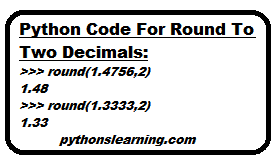Python Code For Round To Two Decimals

## python code for round to two decimals

we know that Python round() function requires two arguments.

• First is the number to be rounded.
• And Second argument decides the number of decimal places to which it is rounded.

So to round the number to 2 decimals, give second argument as 2. If third digit after decimal point is greater than 5, then last digit is increased by 1 position .

``````
>>> round(1.4756,2)
1.48
>>> round(1.3333,2)
1.33``````

### several ways to round decimal digits in python :

1. Round() function use to rounds decimal places up and down. This makes 3.6578 into 3.66 and 6.62392 into 6.62.
2. To round decimal places up we can to also use a custom function. i.e. 4.343 becomes 4.35.
3. Similar to prev.round decimal places down we use a custom function. i.e. turns 1.348 into 1.34.

#### Python Code For Round To Two Decimals on Trinket :

Summary :